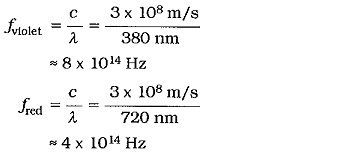## Light Units:

The total light energy output, or luminous fluxs), from a source can be measured in milliwatts (mW) or in lumens (1m), where 1 Im = 1.496 mW. The luminous intensity (Es) (also termed illuminance) of a Light Units source is defined as the luminous flux density per unit solid angle (or cone) emitting from the source, [see Fig. 20-1(a)]. This is measured in Candelas (cd), where one candela is equal to one lumen per unit solid angle, (assuming a point source that emits light evenly in all directions).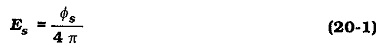The light intensity (EA) on an area at a given distance from the source is determined from the surface area of a sphere surrounding the source, [Fig. 20-1(b)]. At a distance of r meters, the luminous flux is spread over a spherical area of 4 π r2 square meters, so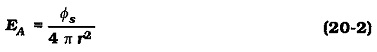When the total flux is expressed in lumens, Eq. 20-2 gives the luminous intensity in lumens per square meter (lm/m2), also termed lux (1x). Comparing Eq. 20-2 to Eq. 20-1, it is seen that the luminous intensity per unit area at any distance r from a point source is determined by dividing the source intensity by r2.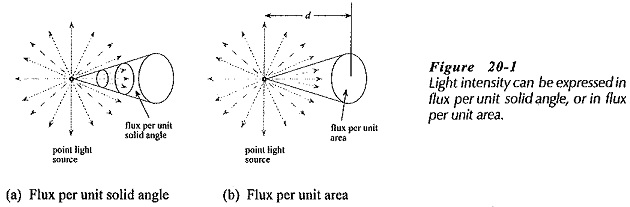Luminous intensity can also be measured in milliwatts per square centimeter (mW/cm2), or lumens per square foot(1m/ ft2), also known as a foot candle (fc), where 1 fc = 10.764 lx.

The Light Units intensity of sunlight on the earth at noon on a clear day is approximately 107,6401x, or 161 W/m2. The light intensity from a 100 W lamp is approximately 4.8 x 103 cd, (allowing for a 90% lamp efficiency). At a distance of 2 m, this is 1.2 x 103 lx. An indicating lamp with a 3 mcd output can be clearly seen at a distance of several meters in normal room lighting conditions.

Light energy is electromagnetic radiation; it is in the form of electromagnetic waves. So, it can be defined in terms of frequency or wavelength, as well as intensity. Wavelength, frequency, and velocity are related by the equation;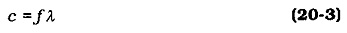where

c = velocity = 3 x 108 m/s for electromagnetic waves

f = frequency in Hz

λ = wavelength in meters

The wavelength of visible light ranges from violet at approximately 380 nm (nanometers) to red at 720 nm. From Eq. 20-3 , the frequency extremes are;